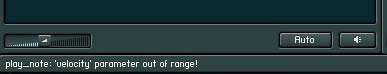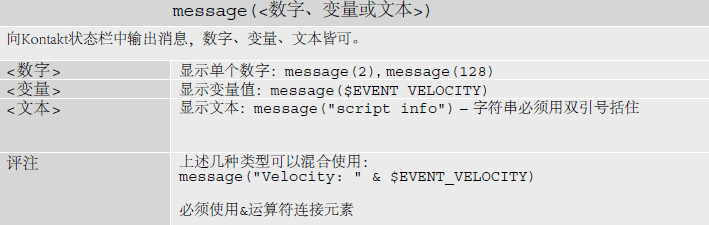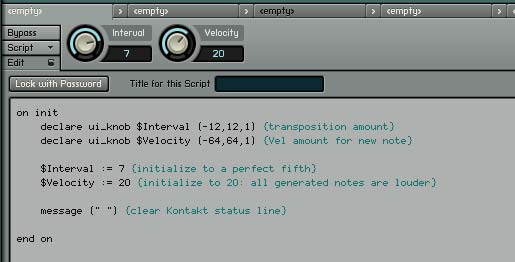• 所有分类
• 宿主
• 工具
• 效果器
• 教程
• 采样器
• 音源
• 预设采样
• 工程模板
• 乐谱

# Kontakt Script官方教程 – 调试错误

[隐藏]

## 调试与自定义：完成脚本

```on init
declare ui_knob \$Interval (-12,12,1)
declare ui_knob \$Velocity (-64,64,1)
\$Interval := 7
\$Velocity := 20
end on

on note
play_note(\$EVENT_NOTE+\$Interval,\$EVENT_VELOCITY+\$VELOCITY,0,-1)
end on``````on init
declare ui_knob \$Interval (-12,12,1)
declare ui_knob \$Velocity (-64,64,1)
\$Interval := 7
\$Velocity := 20
message ("")
end on

on note
if (\$EVENT_VELOCITY+\$VELOCITY > 127)
play_note(\$EVENT_NOTE+\$Interval,127,0,-1)
else
play_note(\$EVENT_NOTE+\$Interval,\$EVENT_VELOCITY+\$VELOCITY,0,-1)
end if
end on```

message函数向Kontakt状态栏中输出消息。完整定义如下：message()函数在检索错误时极为有用，Kontakt状态栏只能显示一条消息，所以展示出来的消息是message()函数生成的最后一条。

`play_note(\$EVENT_NOTE+\$Interval,127,0,-1)`

`play_note(\$EVENT_NOTE + \$Interval, \$EVENT_VELOCITY+\$VELOCITY,0,-1)`

end if标记if语句的结束。

```on init
declare ui_knob \$Interval (-12,12,1) {transposition amount}
declare ui_knob \$Velocity (-64,64,1) {Vel amount for new note}
\$Interval := 7 {initialize to a perfect fifth}
\$Velocity := 20 {initialize to 20: all generated notes are louder}
message (" ") {clear Kontakt status line}
end on

on note
{check if Vel is higher than 127}
if (\$EVENT_VELOCITY+\$VELOCITY > 127)
play_note(\$EVENT_NOTE+\$Interval,127,0,-1)
else
{check if Velocity is lower than 1}
if (\$EVENT_VELOCITY+\$VELOCITY < 1)
play_note(\$EVENT_NOTE+\$Interval,1,0,-1)
else
play_note(\$EVENT_NOTE+\$Interval,\$EVENT_VELOCITY+\$VELOCITY,0,-1)
end if
end if
end on``````on init
{----- GUI elements -----}
declare ui_knob \$Interval (-12,12,1) {transposition amount}
declare ui_knob \$Velocity (-64,64,1) {Vel amount for new note}
{----- Inits -----}
\$Interval := 7 {initialize to a perfect fifth}
\$Velocity := 20 {initialize to 20: all generated notes are louder}
{----- Recall -----}
make_persistent (\$Interval) {save the state of the knob Interval}
make_persistent (\$Velocity) {save the state of the knob Velocity}
message ("") {clear Kontakt status line}
end on

on note
{check if Vel is higher than 127}
if (\$EVENT_VELOCITY+\$VELOCITY > 127)
play_note(\$EVENT_NOTE+\$Interval,127,0,-1)
else
{check if Velocity is lower than 1}
if (\$EVENT_VELOCITY+\$VELOCITY < 1)
play_note(\$EVENT_NOTE+\$Interval,1,0,-1)
else
play_note(\$EVENT_NOTE+\$Interval,\$EVENT_VELOCITY+\$VELOCITY,0,-1)
end if
end if
end on```

 make_persistent(<变量名>) 加载文件后记住变量值。

## 总结

if…else…end if的作用是在不同条件下运行不同指令。message()函数在Kontakt状态栏输出消息，可以显示数字、变量值和文本。make_persistent()函数将变量值保存进文件中。